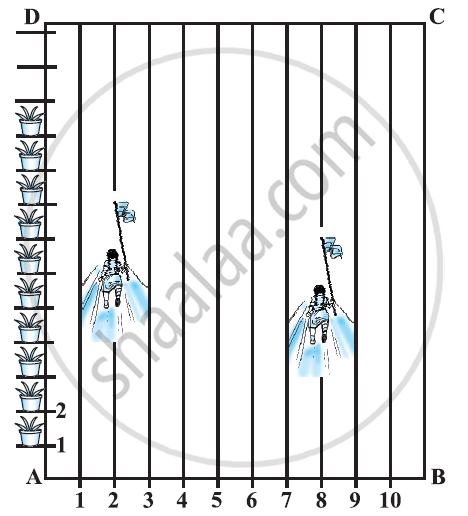# To conduct Sports Day activities, in your rectangular shaped school ground ABCD, lines have been drawn with chalk powder at a distance of 1 m each. 100 flower pots have been placed at a distance of 1 m from each other along AD, as shown in the following figure. - Mathematics

To conduct Sports Day activities, in your rectangular shaped school ground ABCD, lines have been drawn with chalk powder at a distance of 1 m each. 100 flower pots have been placed at a distance of 1 m from each other along AD, as shown in the following figure. Niharika runs 1/4 th the distance AD on the 2nd line and posts a green flag. Preet runs 1/5

th the distance AD on the eighth line and posts a red flag. What is the distance between both the flags? If Rashmi has to post a blue flag exactly halfway between the line segment joining the two flags, where should she post her flag?#### Solution

It can be observed that Niharika posted the green flag at 1/4th of the distance AD i.e., (1×100/4) m = 25m from the starting point of 2nd line. Therefore, the coordinates of this point G is (2, 25). Similarly, Preet posted red flag at 1/5 of the distance AD i.e., (1×100/5) m = 20m from the starting point of 8th line. Therefore, the coordinates of this point R are (8, 20).

Distance between these flags by using distance formula = GR

= sqrt((8-2)^2+(25-20)^2) = sqrt(36+25) = sqrt61m

The point at which Rashmi should post her blue flag is the mid-point of the line joining these points. Let this point be A (xy).

x =(2+8)/2, y =(25+20)/2

x = 10/2 = 5, y = 45/2 = 22.5

Hence A(x,y) = (5,22.5)

Therefore, Rashmi should post her blue flag at 22.5m on 5th line.

Concept: Section Formula
Is there an error in this question or solution?
Chapter 7: Coordinate Geometry - Exercise 7.2 [Page 167]

#### APPEARS IN

NCERT Class 10 Maths
Chapter 7 Coordinate Geometry
Exercise 7.2 | Q 3 | Page 167

Share